# Earthquake data and Benford’s Law

Much has been written about Benford's Law, that weird phenonmenon where if you have a naturally occuring set of numerical data, 30% of the numbers will begin with 1, 18% will begin with 2, 12% will begin with 3, and so on. You might expect the distribution of leading digits to be uniformly distributed, but no, that just isn't the case. 60% of the time the leading digit is 1, 2, or 3. Though it may seem like some curious mathematical anomaly, Benford's Law has been used to detect fraud in elections and accounting.

In this post let's use R to verify that earthquake data obtained from the USGS does indeed follow Benford's Law. On May 28, 2016, I downloaded data for all earthquakes in the past 30 days. The data has a number of fields, including earthquake magnitude, depth of the earthquake, location, time and many others. Benford's Law says those numbers should follow a particular distribution.

The formula for Benford's Law is as follows:

$P(d) = \log_{10} \left(1+\frac{1}{d}\right)$

That says the probability that a digit d occurs as the first number is equal the log base 10 of 1 + 1/d. We can quickly generate these for d = 1 – 9 in R:

log10(1 + (1/(1:9)))

##  0.30103000 0.17609126 0.12493874 0.09691001 0.07918125 0.06694679
##  0.05799195 0.05115252 0.04575749


And we can make a quick plot as follows:

barplot(log10(1 + (1/(1:9))), names.arg = 1:9, main = "Benford's Law")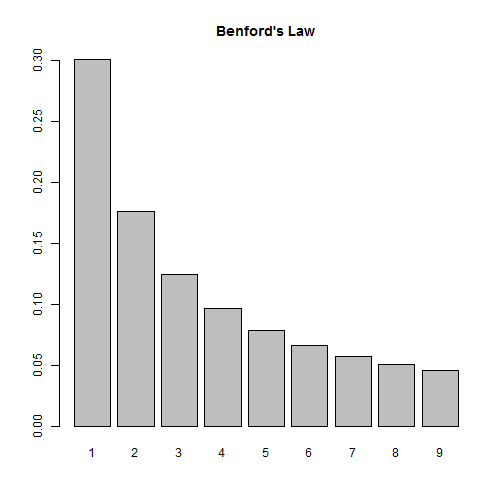So according to this law, if we look at the distribution of first digits in our earthquake data, we should see them closely follow this distribution. Let's find out!

First we need to import the data. Thanks to the USGS, this data comes ready for analysis. All we need to do is read it into R:

dat <- read.csv("all_month.csv")
nrow(dat)

##  8584


Over 8500 earthquakes in the past 30 days! A quick look at the magnitude shows us most of them are very small:

summary(dat$mag)  ## Min. 1st Qu. Median Mean 3rd Qu. Max. NA's ## -1.900 0.790 1.280 1.526 1.930 7.200 20  This also tells us we have some negative numbers (?) as well as some missing data, NA's. We also have numbers that start with 0 and have a decimal, such as 0.79. (In this case, Benford would say the number “starts” with a 7, not a 0.) This means when we determine the leading digits, we'll need to ignore negative signs, leading 0s, decimals and missing values. Let's investigate at the mag column. First we remove the NA's. Below is.na(dat$mag) generates a logical vector of TRUE and FALSE values. Adding an ! in front reverses the TRUE and FALSE values. Finally inserting the result in subsetting brackets returns only those values that are TRUE, ie not missing.

digits <- dat$mag[!is.na(dat$mag)]


Next we extract the first digits. To help with this I use two R packages, magrittr and stringr. The magrittr package allows us to “chain” commands together with the %>% operator. The stringr package provides the str_extract function that allows us to extract phrases that follow a certain pattern. So what we have below can be translated as follows:

• take the absoluet value of digits (to get rid of negative signs)
• convert digits to character (so we can use the next two functions)
• extract anything that is not a “0” or a “.” (We express this a regular expression: "[^0\\.]")
• extract the first digit (starting and ending at position 1)
library(magrittr)
library(stringr)
digits <- abs(digits) %>%
as.character() %>%
str_extract(pattern = "[^0\\.]") %>%
substr(1,1)


As an extra precaution, we then convert digits to a factor and set levels to be all digits 1 through 9. This ensure all digits are represented in subsequent calculations.

digits <- factor(digits, levels = 1:9) # ensure all digits represented


And finally we tally the first digits and calculate proportions:

table(digits) %>% prop.table()

## digits
##          1          2          3          4          5          6
## 0.42800141 0.17134139 0.05738763 0.09447248 0.05809177 0.03990142
##          7          8          9
## 0.04459570 0.05304542 0.05316277


We see 1 appearing a lot more often, but it's hard to tell how the other digits compare to Benford's Law. Let's put both distributions on the same graph. To do this, I went ahead and created a function that will allow us to check any vector of numbers against Benford's Law. Let's load the function and see how it works, then we'll break it down and explain it.

library(ggplot2)
compareBenford <- function(d){
digits <- d[!is.na(d)]
digits <- substr(stringr::str_extract(as.character(abs(digits)), pattern = "[^0\\.]"),1,1)
digits <- factor(digits, levels = 1:9) # ensure all digits represented
depth <- prop.table(table(digits))
ben <- log10(1 + (1/(1:9)))
dat2 <- data.frame(ben, depth)
names(dat2) <- c("Benford","Digit",deparse(substitute(d)))
dat2L <- reshape2::melt(dat2,id.vars="Digit", variable.name = "Type", value.name = "Frequency")
ggplot(dat2L, aes(x=Digit, y=Frequency, fill=Type)) +
geom_bar(stat = "identity", position = "dodge")
}

compareBenford(dat$mag)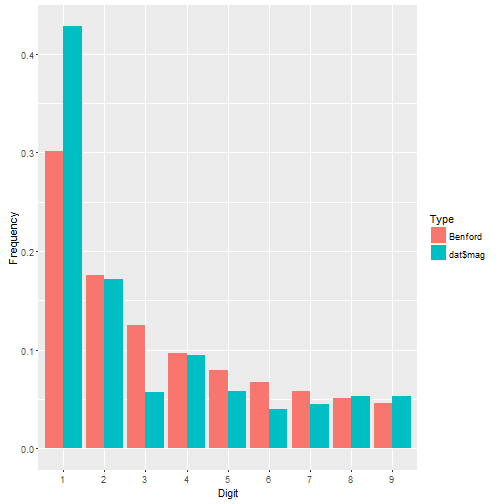We see dat$mag has more 1's than we might expect and fewer 3's, but otherwise seems to follow the distribution pretty closely. Let's check out earthquake depth.

compareBenford(dat$depth)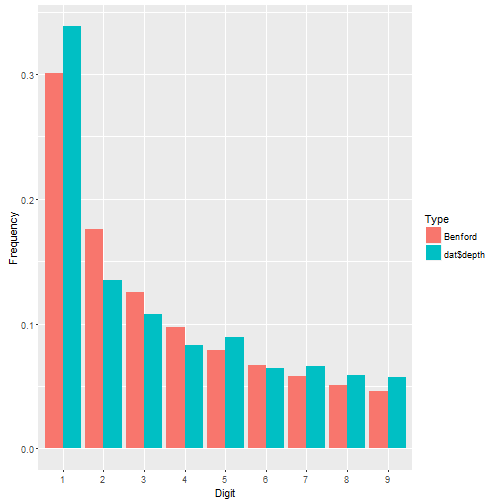This appears to fit even better. About the function: • the first four lines are what we did before. Notice I went ahead and nested the functions instead of using magrittr. That's how I originally coded it before deciding to write a blog post. Then I decided to break out magrittr for the fun of it. • After that I calculate Benford's proportions. • Then I put both sets of proportions in a data frame. • Then I change the names of the data frame. Notice there are three names to change, not two. Why? The depth vector is actually a table. When it gets pulled into a data frame, two columns are produced: the table cells and the table names. The names are the digits 1 – 9. Notice I also use deparse(substitute(d)) to name the first-digit proportions in the data frame. This ensures that whatever vector I give my function, the name of it will be the vector itself. So if I give it dat$mag, the column name of the first-digit proportions will be dat$mag. • Next I reshape the data using the melt function in the reshape2 package. This puts all the proportions in one column called Frequency and the type of proportion in another column called Type. I still have my Digit column that indicates which proportion goes with which digit. Having my data in this form allows me to use ggplot to map fill color to Type. • Finally I create the graph. I set Digit on the x-axis, Frequency on the y-axis, and map Type to the fill color. The geom_bar function says draw a bar plot. The stat = "identity" and position = "dodge" arguments say set the height of the bars to the actual values and “dodge” them so they're next to one another. Let's look at ALL numeric columns in the earthquakes data. We need to identify all numeric columns and pull them into a vector for our function to work. Here's how I do it: theCols <- sapply(dat, class) %in% c("numeric","integer") numCols <- names(dat)[theCols] allDigits <- unlist(dat[,numCols]) compareBenford(allDigits)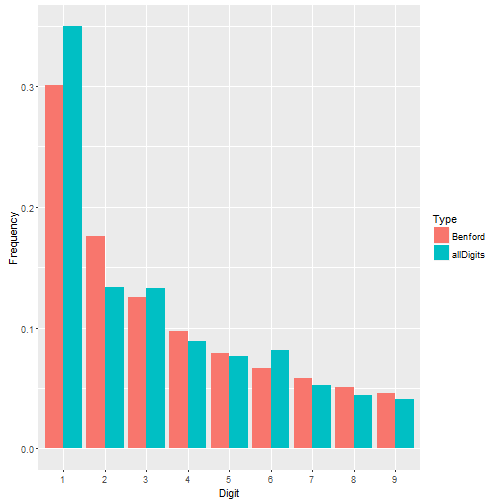Not a bad fit. Benford's Law seems to work just fine for the earthquake data. But what about data where Benford's Law doesn't work? Recall that Benford's Law applies to most naturally occuring sets of numbers. Not all. Take the iris data that come with R. These are measurements of 50 flowers from each of 3 species of iris. This is a small data set looking at very specific measurements. It doesn't seem to follow Benford's Law very well. irisDigits <- unlist(iris[,1:4]) compareBenford(irisDigits)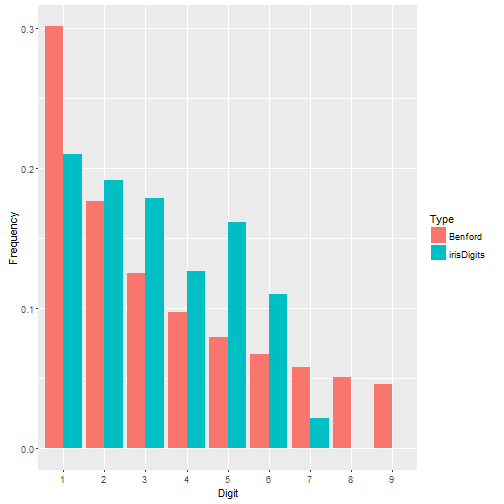# Recreating a graph from a NCHS data brief I was reading this sobering article about the rise of suicide rates in the United States and decided to read the NCHS data brief on which it was based. I noticed the source data was freely available online through the CDC Wonder databases, specifically the Detailed Mortality database, and figured I would take a look. One graph that caught my eye was Figure 2, which showed that suicide rates for females were higher in 2014 than in 1999 for all age groups under 75 years.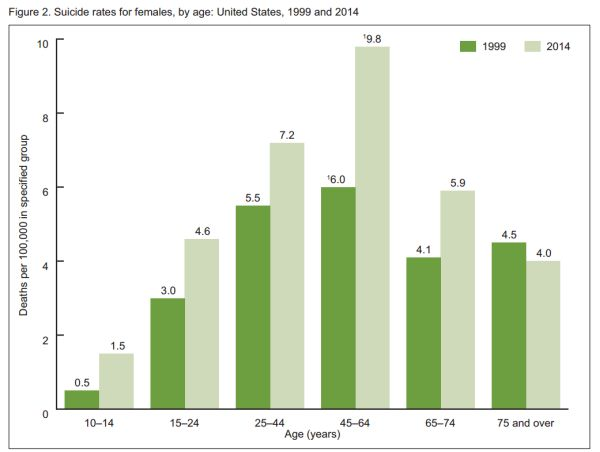That's a very sad and concerning graph. What happened in the last 15 years? I have no theories about the increase in suicide rates, but I do admire the CDC statisticians who tackle this kind of gut-wrenching data, day in and day out. Let's join them for a moment and recreate this graph in R. First we need to get the data from the Detailed Mortality database. Instead of directly downloading raw data, we submit a query for the data we want. There is a 7-step form to complete. Step 1: Group by ICD-10 113 Cause List, Cause of death, Age Groups, Gender, and Year. This uses all available pull-down lists. Step 2: leave as is. (ie, all US states) Step 3: leave as is. (ie, all genders, ages, and races) Step 4: select 1999 and 2014 using Ctrl + Click to select both years Step 5: leave as is. (ie, all weekdays, autopsies, and places of death) Step 6: select the ICD-10 113 Cause List radio button and select “Intentional self-harm (suicide) (*U03,X60-X84,Y87.0)” Step 7: click Send. The results should appear in your web browser. If everything looks good, click the Export button to download a tab-delimited file of the results. It will have a .txt extenstion. Now that we have our data, we can go to R and get to work. Before we read in the data we should open it in a text editor and see what we got. We have a header row, so that's good. If we scroll down, we'll see there's about 70 lines of metadata. This is data about our data, such as where it came from, when we downloaded it, etc. We don't want that in our data frame, but we should save it with our data frame as an attribute. To start we'll use readLines to read in the data one line at a time. I chose to do this as a way to programmatically figure out where the metadata begins. I noticed it begins after a divider of three dashes (“—”), so I use grep in combination with min to find the first occurence of this divider and save a n. raw <- readLines("Underlying Cause of Death, 1999-2014 (3).txt")  ## Warning in readLines("Underlying Cause of Death, 1999-2014 (3).txt"): ## incomplete final line found on 'Underlying Cause of Death, 1999-2014 ## (3).txt'  n <- min(grep(pattern = "---",raw))  Now I can use n with the nrows argument in the read.delim function to tell it how many rows I want to read in. Notice I have to say n - 2. I have to subtract the header row and the divider row itself. I also specify “Not Applicable” and “Unreliable” as NA values. Finally, take a look at my file name. Yep, it took me a few tries to get the data I wanted. I'm not too proud to admit that. :) dat <- read.delim(file = "Underlying Cause of Death, 1999-2014 (3).txt", header = TRUE, nrows = n-2, na.strings = c("Not Applicable","Unreliable"))  Let's go ahead and save the metadata with our data frame. We can do that with the comment function. We don't need it to create the graph, but it's good practice to preserve this kind of information. To see the metadata after we save, just enter comment(dat). It's not pretty when it's printed to the console, but we have it if we need it. comment(dat) <- raw[n:length(raw)]  Now we need to clean up the data. First order of business is to reorder the age groups. By default, R brought them in as a factor and put the 15-24 age group as the first level. We want that first level to be the 5-14 age group. It's already a factor, but we reorder the levels by making the column a factor again and specifying the levels in the specified order. The original 5th level comes first and then everything else. There may be a better way of doing this, but it works. dat$Ten.Year.Age.Groups.Code <- factor(dat$Ten.Year.Age.Groups.Code, levels = levels(dat$Ten.Year.Age.Groups.Code)[c(5,1,2,3,4,6,7,8,9,10)])


We only need females, so let's subset. I make a new data frame called dat2.

dat2 <- subset(dat, Gender=="Female")


Now if we look at Figure 2 again, the x-axis has 6 age groups, but our data has 9 age groups. So we need to combine a few age groups. Specifically we need to combine the “25-34” and “35-44” groups into “25-44”, combine the “45-54” and “55-64” groups into “45-64”, and the “75-84” and “85+” into “75+”. That's not too bad. We just reset the levels.

levels(dat2$Ten.Year.Age.Groups.Code) <- c("5-14", "15-24", "25-44", "25-44", "45-64", "45-64", "65-74", "75+", "75+", "NS")  Since we have 6 age groups instead of 9, we need to combine death totals for the three new age groups. We also need to combine death totals for all the various causes of deaths. We can do that with aggregate. Below we sum Deaths by Ten.Year.Age.Groups.Code and Year. This returns a new data frame that I call dat3. (dat3 <- aggregate(Deaths ~ Ten.Year.Age.Groups.Code + Year, data=dat2, sum))  ## Ten.Year.Age.Groups.Code Year Deaths ## 1 5-14 1999 50 ## 2 15-24 1999 575 ## 3 25-44 1999 2359 ## 4 45-64 1999 1868 ## 5 65-74 1999 420 ## 6 75+ 1999 469 ## 7 5-14 2014 151 ## 8 15-24 2014 990 ## 9 25-44 2014 3018 ## 10 45-64 2014 4195 ## 11 65-74 2014 828 ## 12 75+ 2014 477 ## 13 NS 2014 1  Next I need to calculate “deaths per 100,000” for each age group. We do this by dividing Deaths by the age group population and multiplying by 100,000. I need to add the age group populations to dat3 to do this. First I need to sum the populations for my new age groups. I can use aggregate again with dat2, but I need to remove duplicate rows of age group population values before I do this. I decided to save the de-duped data frame to tmp and not overwrite dat2 tmp <- subset(dat2, select = c("Year", "Ten.Year.Age.Groups.Code", "Population")) tmp <- tmp[!duplicated(tmp),] (dat4 <- aggregate(Population ~ Ten.Year.Age.Groups.Code + Year, data=tmp, sum))  ## Ten.Year.Age.Groups.Code Year Population ## 1 5-14 1999 19908282 ## 2 15-24 1999 18860184 ## 3 25-44 1999 42614694 ## 4 45-64 1999 31011068 ## 5 65-74 1999 10125424 ## 6 75+ 1999 10371906 ## 7 5-14 2014 20161424 ## 8 15-24 2014 21456371 ## 9 25-44 2014 41900194 ## 10 45-64 2014 42789506 ## 11 65-74 2014 14049245 ## 12 75+ 2014 11842674  Now I'm ready to merge dat3 and dat4 so I have Deaths and Populations in one data frame. Before I do that I remove the row with age group of “NS” which means “Not Stated”. We don't need that row. dat3 <- subset(dat3, Ten.Year.Age.Groups.Code != "NS") dat5 <- merge(dat3,dat4,by = c("Year","Ten.Year.Age.Groups.Code"))  And finally we can calculate Rate. I decided to also sort the data frame as well. dat5$Rate <- round(dat5$Deaths/dat5$Population * 100000,1)
(dat5 <- dat5[order(dat5$Ten.Year.Age.Groups.Code, dat5$Year),])

##    Year Ten.Year.Age.Groups.Code Deaths Population Rate
## 4  1999                     5-14     50   19908282  0.3
## 10 2014                     5-14    151   20161424  0.7
## 1  1999                    15-24    575   18860184  3.0
## 7  2014                    15-24    990   21456371  4.6
## 2  1999                    25-44   2359   42614694  5.5
## 8  2014                    25-44   3018   41900194  7.2
## 3  1999                    45-64   1868   31011068  6.0
## 9  2014                    45-64   4195   42789506  9.8
## 5  1999                    65-74    420   10125424  4.1
## 11 2014                    65-74    828   14049245  5.9
## 6  1999                      75+    469   10371906  4.5
## 12 2014                      75+    477   11842674  4.0


A quick comparison of our rates with those in Figure 2 shows that we're in agreement. Now it's time to re-create the graph. For this I chose to use ggplot2. To match the colors, I uploaded a picture of the original graph to this wonderful site and figured out the shades of green were #6D9F40 (dark green) and #CFDABA (light green).

library(ggplot2)
ggplot(dat5, aes(x=Ten.Year.Age.Groups.Code, y=Rate, fill=factor(Year))) +
geom_bar(stat="identity", position = "dodge") +
labs(x="Age (years)",y="Deaths per 100,000 in specified group") +
scale_fill_manual("Year", values = c("#6D9F40","#CFDABA")) +
annotate(geom = "text", x = (rep(1:6,each=2) + c(-1,1)*0.22),
y = dat5$Rate + 0.2, label = sprintf("%.1f", dat5$Rate)) +
theme_bw() +
scale_y_continuous(breaks=seq(0,10,2))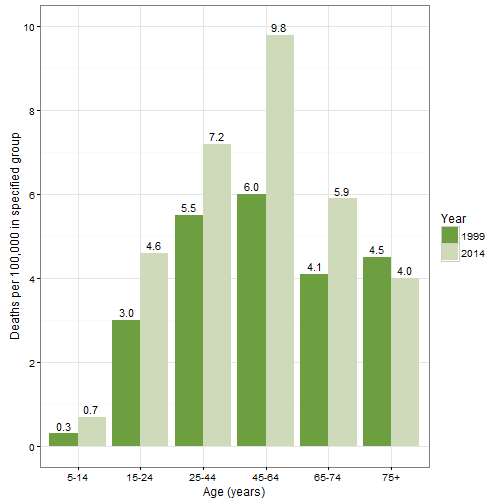The first line defines the aesthetics. I want Year to be treated like a categorical variable, so I call it with factor. The next lines says, “don't count the rows to create the bar graph, use the actual values of Rate (ie, identity).” It also says “dodge”“ the Years so they're next to each other (instead of stacked). The third line labels the axes. The fourth line titles the legend and defines the colors. The annontate line adds the counts to the plot. That took some trial and error! The x and y values are the coordinates. The label is the Rate values rendered as character with one decimal place. The theme_bw() line is an easy way to get rid of the default grey background and the last line relabels the y axis.

# Review of R Graphs Cookbook

I was recently invited to review the second edition of the R Graphs Cookbook by Jaynal Abedin and Hrishi V. Mittal (Packt Publishing). This is not to be confused with the R Graphics Cookbook by Winston Chang (O’Reilly Media). I own the latter and refer to it on a regular basis. I was curious to see how the R Graphs Cookbook would compare to it.

The book has 15 chapters. The first 8 cover how to do traditional graphs such as scatter plots, line graphs, histograms, box plots and the like along with extensive coverage of tweaking graphical parameters. Chapters 9 – 14 cover special topics like heat maps, geographical maps, lattice and ggplot2. The final chapter presents several recipes for finalizing graphs for publication.

Each “recipe” follows a template of first showing How to Do It followed by a How it Works section that walks you through the code. There’s also a There’s More section that offers extra tips or suggestions and finally a See Also section that refers you to similar recipes. The recipes frequently use sample data sets that need to be downloaded from the Packt Publishing site. Otherwise they tend to use data sets included with the base R datasets package or randomly generated data. Each recipe includes the graph that it produces in color, at least they do in the the PDF version I reviewed. (I don’t know if the paperback includes color. For the asking price of \$46 on Amazon I hope it does.)

My overall impression of the book is positive. The recipes are practical, the explanations of code are clear, and there are several pointers to useful R packages.The chapters that really stood out to me (ie, chapters I could see myself using) were the coverage of graphical parameters in chapter 3, working with map and GIS data in chapter 10, and preparing graphs for publication in chapter 15.

But the book isn’t perfect. My biggest complaint is the book datasets. They’re in a folder structure that doesn’t always match the book’s contents. For example there is a health expenditure dataset in the Chapter 3 folder that is not used until Chapter 4. As another example, the recipe for choosing plot symbols and sizes asks us to load the “cityrain.csv” data “that we used in the first chapter.” But it’s not used in the first chapter, it’s used it in the second chapter. But in this case the dataset is actually in the Chapter 2 folder! I frequently found myself setting my working directory to use a chapter’s dataset only to find the dataset wasn’t there. All this could have been avoided by just lumping all data into a single folder. Or perhaps by making an R package that contains the book’s datasets as the author of the R Graphics Cookbook has done.

Another head-scratcher is the introduction to the grid package in the first section of Chapter 1. The section is titled “Base graphics using the default package”, yet grid is presented as the base graphics package. It’s not. The base R graphics package is the graphics package. The authors clearly know a great deal about creating R graphics, but I don’t understand their reasoning for presenting the grid package as the default package for graphs.

There are a few recipes that I think could be improved. The “Formatting log axes” recipe simply plots$10^1$ through$10^5$ with the log argument set to “y”. Why not use one of the book’s datasets and show a before and after graph to demonstrate how the axis changes with log formatting? For example:

 > metals <- read.csv("Chap 3/Data Files/metals.csv") > par(mfrow=c(1,2)) > plot(Ba~Cu,data=metals,xlim=c(0,100), main="Before y-axis\n log transformation") > plot(Ba~Cu,data=metals,xlim=c(0,100), log="y", main="After y-axis\nlog transformation") > par(mfrow=c(1,1))The “Creating bar charts with vertical error bars” recipe in chapter 6 creates error bars by multiplying the plotted values by 0.95 and 1.05. Why not show how to plot actual standard error bars? In fact they conclude the recipe by saying “In practice, scaled estimated standard deviation values or other formal estimates of error would be used to draw error bars instead of a blanket percentage error as shown here.” To their credit, the authors do show how to create conventional standard error bars later on in the ggplot2 chapter. At the very least it seems like the recipe in chapter 6 should have a See Also section that points readers to the ggplot2 recipe.

One other recipe I thought could be better was “Setting graph margins and dimensions”. It tells you how to do it but doesn’t actually demonstrate it. It would have been nice to see the effect of changing the various parameters. In fact I’m still not sure how the fin and pin par() arguments work. Of course the authors go on to say “it is better to use mar or mai” instead of fin and pin, which I suppose is nice since I know how mar and mai work. But then why mention fin and pin in the first place?

While I’m on the subject of setting graphics parameters I noticed the authors never explicitly explain how to restore initial par() values by saving the result of par() when making changes. For example,

> oldpar <- par(col=4, lty=2) … plotting commands … > par(oldpar)

They do it once in chapter 1 when demonstrating trellis graphs but they don’t explain what it’s doing or why it’s there. I really believe that should be a separate recipe in chapter 3, “Beyond the Basics – Adjusting Key Parameters”.

Some of the recipes I really liked were “Setting fonts for annotations and titles”, “Using margin labels instead of legends for multiple-line graphs” and “Showing the number of observations” in the axis labels of box plots. Those three are golden. The recipe for “Graph annotation with ggplot” is also quite useful. And I thoroughly enjoyed working through the “Data Visualization Using Lattice” chapter. I had never used Lattice before and found this to be an excellent tutorial.

As I mentioned earlier, I own and make regular use of O’Reilly’s R Graphics Cookbook. How does Packt’s R Graphs Cookbook compare? The main difference is ggplot2. The O’Reilly book is almost exclusively devoted to ggplot2. In fact if not for the Miscellaneous Graphs chapter near the end it could very easily be called the ggplot2 Cookbook. The R Graphs Cookbook on the other hand is rooted in base R graphics. ggplot2 gets introduced briefly in chapters 1 and 4 before getting its own treatment in chapter 12. If you’re looking for a ggplot2 reference, O’Reilly’s R Graphics Cookbook is hands-down the best choice. But if you want a reference for base R graphics, then the R Graphs Cookbook is the better of the two.